# Geospatial Big Data Processing with Python: Detecting Green Roofs in Toronto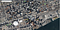Detecting Green Roofs in Toronto

# Image Processing: 1.3 Billion Points

`plt.figure(figsize=(12, 8))data.plot()# plotting takes time due to large file size`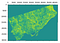Raster
`df = data.to_pandas()`
`# Converting raster to csv. this process takes time.from osgeo import gdalfrom osgeo import gdalconstInRaster = r”downloads\ndvi_merged\merged.tif”OutCSV = r"D:\downloads\ndvi_merged\test2.csv"# open the raster and get propertiesds = gdal.OpenShared(InRaster,gdalconst.GA_ReadOnly)GeoTrans = ds.GetGeoTransform()ColRange = range(ds.RasterXSize)RowRange = range(ds.RasterYSize)rBand = ds.GetRasterBand(1) # first bandnData = rBand.GetNoDataValue()if nData == None: nData = -9999else: print(“NoData value is {0}”.format(nData))HalfX = GeoTrans / 2HalfY = GeoTrans / 2with open(OutCSV,’w’) as CSVwrite: for ThisRow in RowRange: RowData = rBand.ReadAsArray(0,ThisRow,ds.RasterXSize,1) for ThisCol in ColRange: if RowData[ThisCol] != nData: if RowData[ThisCol] > 0: X = GeoTrans + ( ThisCol * GeoTrans ) Y = GeoTrans + ( ThisRow * GeoTrans )  X += HalfX Y += HalfY CSVwrite.write(‘{0},{1},{2}\n’.format(X,Y,RowData[ThisCol]))`
`size= os.path.getsize(r”D:\downloads\ndvi_merged\test2.csv”)print(‘File size: ‘ + str(round(size / (1024 * 1024 * 1024), 3)) + ‘ Gigabytes’)`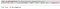CSV File Size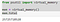Desktop memory

# Dask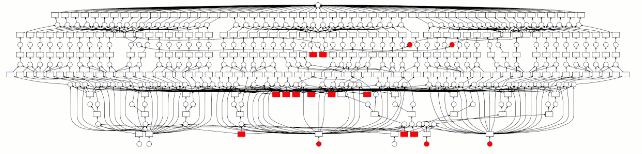Dask: Scale up to clusters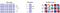https://dask.org/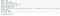Reading CSV
`df_tmp`
`import timestart = time.time()import dask.dataframe as ddimport multiprocessingimport dask_geopandas as dg# dask’s read_csv takes no time at all!ddf = dd.read_csv(r”D:\downloads\ndvi_merged\test2.csv”, names=[‘lng’, ‘lat’, ‘ndvi’])end = time.time()print(end — start)#0.3410015106201172`
`ddf[[‘lng’,’lat’,’ndvi’]]=ddf[[‘lng’,’lat’,’ndvi’]].astype(‘float32’)`
`# get first partitionpart_0= ddf.get_partition(0)part_0.tail()`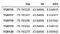The first partition of dataframe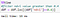Filtering DataFrame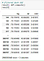Filtered DataFrame

# Building Data

`# download building dataimport zipfileimport iofrom pyproj import Proj, CRS, transformurl = ‘https://ckan0.cf.opendata.inter.prod-toronto.ca/download_resource/09197dd4-f440-49c0-acc5-41e2585ef9f7'local_path = ‘./tmp’print(‘Downloading zipped shapefile…’)r = requests.get(url)z = zipfile.ZipFile(io.BytesIO(r.content))print(“Done”)z.extractall(path=local_path) # extract to tmp folderfilenames = [y for y in sorted(z.namelist()) for ending in [‘dbf’, ‘prj’, ‘shp’, ‘shx’] if y.endswith(ending)] print(filenames)shp= gpd.read_file(r’./tmp/3DMassing_2019_WGS84.shp’)shp.head(5)`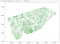Toronto Building Data

# Spatial Join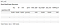Spatially joined Dask DataFrame
`part_0= ddf_out.get_partition(0)part_0.tail()`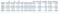Spatially joined NDVI data
`points = ddf_out.compute()crs = CRS(“EPSG:3857”)points_gdf = gpd.GeoDataFrame(points, crs = crs, geometry=’geom’)points_gdf.head(10)`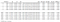NDVI point data
`#groupby building_id and count ndvi values by  using dask%timeresult = ddf_out.groupby(ddf_out.building_id).ndvi.count().compute()`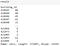Grouped NDVI Data by Buildings
`merged = gpd.GeoDataFrame(pd.merge(result.to_frame(), shp, left_index=True, right_on='building_id'))merged`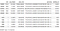Building NDVI data
`ax = points_gdf.plot(figsize=(15, 15), markersize =1)shp.plot(ax=ax,edgecolor=’cyan’, facecolor = ‘white’, linewidth =1)points_gdf.plot(ax = ax,color=’green’, markersize =1)ax.set(xlim=(-8849000, -8842000), ylim=(5421000, 5423500))`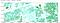Building Polygons with NDVI data

# Zonal Statistics and Spatial Join in ArcPro

`%%timeimport arcpyimport pandas as pdfrom arcgis.features import GeoAccessor, GeoSeriesAccessorfrom arcgis.features import SpatialDataFramefrom arcpy import envimport numpy as npimport os,sysarcpy.ia.ZonalStatisticsAsTable(“building4326”, “FID”, “ndvi_merged_4326.tif”, r”C:\Users\gis\Documents\ArcGIS\Projects\deep\deep.gdb\ZonalStat”, “DATA”, “ALL”, “CURRENT_SLICE”, 90, “AUTO_DETECT”)ZonalStat= arcpy.management.AddJoin(“building4326”, “FID”, “ZonalStat”, “FID”, “KEEP_ALL”)def table_to_data_frame(in_table, input_fields=None, where_clause=None):    """Function will convert an arcgis table into a pandas dataframe with an object ID index, and the selected    input fields using an arcpy.da.SearchCursor."""    OIDFieldName = arcpy.Describe(in_table).OIDFieldName    if input_fields:        final_fields = [OIDFieldName] + input_fields    else:        final_fields = [field.name for field in arcpy.ListFields(in_table)]    data = [row for row in arcpy.da.SearchCursor(in_table, final_fields, where_clause=where_clause)]    fc_dataframe = pd.DataFrame(data, columns=final_fields)    fc_dataframe = fc_dataframe.set_index(OIDFieldName, drop=True)    return fc_dataframezonalstat = table_to_data_frame("building4326")zonalstat.head(5)#Wall time: 4min 28s`

# GeoPandas and RasterStats

`%%timefrom rasterstats import zonal_statsbldg1 =gpd.read_file(r"C:\Users\gis\Documents\tmp\building4326.shp")bldg1['count'] = pd.DataFrame(    zonal_stats(        vectors=bldg1['geometry'],         raster=r"D:\downloads\ndvi_merged\ndvi_merged_4326.tif",         stats='count'    ))['count']`
`filtered = merged.nlargest(1000,[‘ndvi’,’avg_height’,’poly_area’])filtered.head(20)`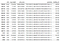Filtered Buildings
`shp_165816 = shp.loc[(shp[‘building_id’]==165816)] points_165816 = points_gdf.loc[(points_gdf[‘building_id’]==165816)]ax = points_165816.plot(figsize=(15, 15), markersize =1)shp_165816.plot(ax=ax,edgecolor=’red’, facecolor = ‘white’, linewidth =1)points_165816.plot(ax = ax,color=’green’, markersize =1)`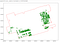The First Building with Green Roof
`def embed_map(m): from IPython.display import IFrame m.save(‘index.html’) return IFrame(‘index.html’, width=’100%’, height=’750px’)m = folium.Map(location=[43.6532, -79.3832], zoom_start=12, tiles=’http://services.arcgisonline.com/arcgis/rest/services/World_Imagery' + ‘/MapServer/tile/{z}/{y}/{x}’, attr=’Ablajan or anything else…’)building = filtered.to_crs(epsg=’4326').to_json()style = {‘fillColor’: ‘#006400’, ‘lineColor’: ‘#228B22’}building = folium.features.GeoJson(building, style_function=lambda x:style)m.add_child(building)results =”C:\\Users\\gis\\Desktop\\”m.save(os.path.join(results, ‘building.html’))embed_map(m)`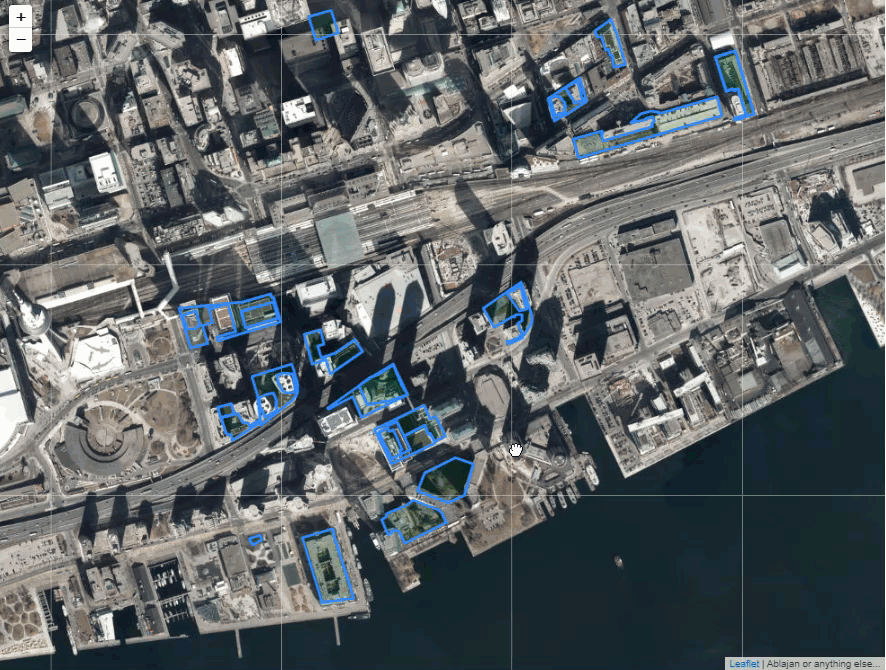Plotting Green Roofs with Folium

# Machine Learning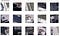Single-Shot Detector (SSD)
`# Load a random image from the images folderIMAGE_DIR=r”C:\dev\timg”file_names = next(os.walk(IMAGE_DIR))image = cv2.imread(os.path.join(IMAGE_DIR, random.choice(file_names)))# # load model weightsmodel_path = r’C:\Users\gis\Downloads\greenroof\gr_cfg20191111T1809\mask_rcnn_gr_cfg_0005.h5'class_names = [‘BG’, ‘greenroof’]model.load_weights(model_path, by_name=True)# Run detectionresults = model.detect([image], verbose=1)# Visualize resultsr = resultsvisualize.display_instances(image, r[‘rois’], r[‘masks’], r[‘class_ids’], class_names, r[‘scores’])`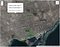Toronto Green Roofs

# Conclusion

## References:

Senior Geospatial Specialist in Toronto

## More from Ablajan Sulaiman

Senior Geospatial Specialist in Toronto